# Tag: artyLast time we did recall Manin’s comparisons between some approaches to geometry over the absolute point $\pmb{spec}(\mathbb{F}_1)$ and trends in the history of art.

In the comments to that post, Javier Lopez-Pena wrote that he and Oliver Lorscheid briefly contemplated the idea of extending Manin’s artsy-dictionary to all approaches they did draw on their Map of $\mathbb{F}_1$-land.

So this time, we will include here Javier’s and Oliver’s insights on the colored pieces below in their map : CC=Connes-Consani, Generalized torified schemes=Lopez Pena-Lorscheid, Generalized schemes with 0=Durov and, this time, $\Lambda$=Manin-Marcolli.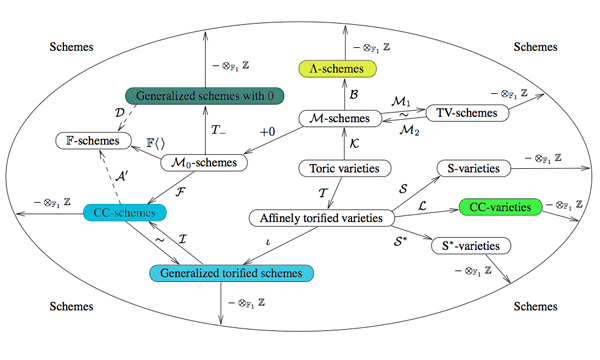Durov : romanticismIn his 568 page long Ph.D. thesis New Approach to Arakelov Geometry Nikolai Durov introduces a vast generalization of classical algebraic geometry in which both Arakelov geometry and a more exotic geometry over $\mathbb{F}_1$ fit naturally. Because there were great hopes and expectations it would lead to a big extension of algebraic geometry, Javier and Oliver associate this approach to romantism. From wikipedia : “The modern sense of a romantic character may be expressed in Byronic ideals of a gifted, perhaps misunderstood loner, creatively following the dictates of his inspiration rather than the standard ways of contemporary society.”

Manin and Marcolli : impressionismYuri I. Manin in Cyclotomy and analytic geometry over $\mathbb{F}_1$ and Matilde Marcolli in Cyclotomy and endomotives develop a theory of analytic geometry over $\mathbb{F}_1$ based on analytic functions ‘leaking out of roots of unity’. Javier and Oliver depict such functions as ‘thin, but visible brush strokes at roots of 1’ and therefore associate this approach to impressionism. Frow wikipedia : ‘Characteristics of Impressionist paintings include: relatively small, thin, yet visible brush strokes; open composition; emphasis on accurate depiction of light in its changing qualities (often accentuating the effects of the passage of time); common, ordinary subject matter; the inclusion of movement as a crucial element of human perception and experience; and unusual visual angles.’

Connes and Consani : cubismIn On the notion of geometry over $\mathbb{F}_1$ Alain Connes and Katia Consani develop their extension of Soule’s approach. A while ago I’ve done a couple of posts on this here, here and here. Javier and Oliver associate this approach to cubism (a.o. Pablo Picasso and Georges Braque) because of the weird juxtapositions of the simple monoidal pieces in this approach.

Lopez-Pena and Lorscheid : deconstructivismTorified varieties and schemes were introduced by Javier Lopez-Pena and Oliver Lorscheid in Torified varieties and their geometries over $\mathbb{F}_1$ to get lots of examples of varieties over the absolute point in the sense of both Soule and Connes-Consani. Because they were fragmenting schemes into their “fundamental pieces” they associate their approach to deconstructivism.

Another time I’ll sketch my own arty-farty take on all this.

No christmas- or new-years family party without heated discussions. Often on quite silly topics.

For example, which late 19th-century bookcharacter turned out to be most influential in the 20th century? Dracula, from the 1897 novel by Irish author Bram Stoker or Sir Arthur Conan Doyle’s Sherlock Holmes who made his first appearance in 1887?

Well, this year you can spice up such futile discussions by going over to Google Labs Books Ngram Viewer, specify the time period of interest to you and the relevant search terms and in no time it spits back a graph comparing the number of books mentioning these terms.

Here’s the 20th-century graph for ‘Dracula’ (blue), compared to ‘Sherlock Holmes’ (red).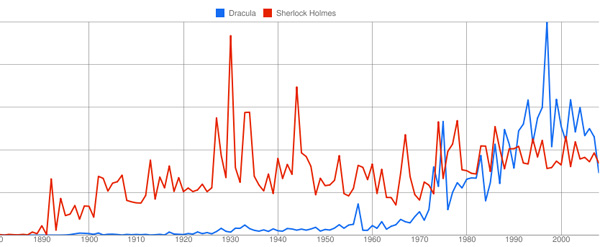The verdict being that Sherlock was the more popular of the two for the better part of the century, but in the end the vampire bit the detective. Such graphs lead to lots of new questions, such as : why was Holmes so popular in the early 30ties? and in WW2? why did Dracula become popular in the late 90ties? etc. etc.

Clearly, once you’ve used Books Ngram it’s a dangerous time-waster. Below, the graphs in the time-frame 1980-2008 for Alain Connes (blue), noncommutative geometry (red), Hopf algebras (green) and quantum groups (yellow).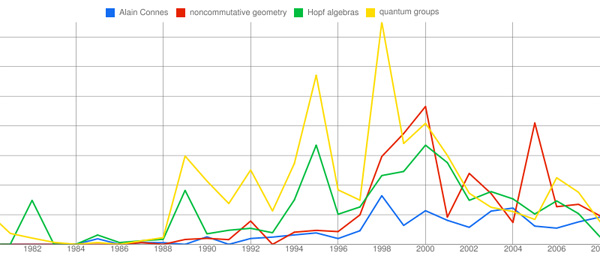It illustrates the simultaneous rise and fall of both quantum groups and Hopf algebras, whereas the noncommutative geometry-graph follows that of Alain Connes with a delay of about 2 years. I’m sure you’ll find a good use for this splendid tool…

Terry Tao is reworking some of his better blogposts into a book, to be published by the AMS (here’s a preliminary version of the book “What’s New?”)

After some thought, I decided not to transcribe all of my posts from last year (there are 93 of them!), but instead to restrict attention to those articles which (a) have significant mathematical content, (b) are not announcements of material that will be published elsewhere, and (c) are not primarily based on a talk given by someone else. As it turns out, this still leaves about 33 articles from 2007, leading to a decent-sized book of a couple hundred pages in length.

If you have a blog and want to turn it into a LaTeX-book, there’s no need to transcribe or copy every single post, thanks to the WPTeX tool. Note that this is NOT a WP-plugin, but a (simple at that) php-program which turns all posts into a bookcontent.tex file. This file can then be edited further into a proper book.

Unfortunately, the present version chokes on LaTeXrender-code (which is easy enough to solve doing a global ‘find-and-replace’ of the tex-tags by dollar-signs) but worse, on Markdown-code… But then, someone fluent in php-regex will have no problems extending the libs/functions.php file (I hope…).

At the moment I’m considering turning the Mathieu-games-posts into a booklet. A possible title might be Mathieumatical Games. Rereading them (and other posts) I regret to be such an impatient blogger. Often I’m interested in something and start writing posts about it without knowing where or when I’ll land. This makes my posts a lot harder to get through than they might have been, if I would blog only after having digested the material myself… Typical recent examples are the tori-crypto-posts and the Bost-Connes algebra posts.

So, I still have a lot to learn from other bloggers I admire, such as Jennifer Ouellette who maintains the Coctail Party Physics blog. At the moment, Jennifer is resident blogger-journalist at the Kavli Institute where she is running a “Journal Club” workshop giving ideas on how to write better about science.

But the KITP is also committed to fostering scientific communication. That’s where I come in. Each Friday through April 26th, I’ll be presiding over a “Journal Club” meeting focusing on some aspect of communicating science.

Her most recent talk was entitled To Blog or Not to Blog? That is the Question and you can find the slides as well as a QuickTime movie of her talk. They even plan to set up a blog for the participants of the workshop. I will surely follow the rest of her course with keen interest!We will see later that the cyclic subgroup $T_6 \subset \mathbb{F}_{p^6}^*$ is a 2-dimensional torus.

Take a finite set of polynomials $f_i(x_1,\ldots,x_k) \in \mathbb{F}_p[x_1,\ldots,x_k]$ and consider for every fieldextension $\mathbb{F}_p \subset \mathbb{F}_q$ the set of all k-tuples satisfying all these polynomials and call this set

$X(\mathbb{F}_q) = { (a_1,\ldots,a_k) \in \mathbb{F}_q^k~:~f_i(a_1,\ldots,a_k) = 0~\forall i }$

Then, $T_6$ being a 2-dimensional torus roughly means that we can find a system of polynomials such that
$T_6 = X(\mathbb{F}_p)$ and over the algebraic closure $\overline{\mathbb{F}}_p$ we have $X(\overline{\mathbb{F}}_p) = \overline{\mathbb{F}}_p^* \times \overline{\mathbb{F}}_p^*$ and $T_6$ is a subgroup of this product group.

It is known that all 2-dimensional tori are rational. In particular, this means that we can write down maps defined by rational functions (fractions of polynomials) $f~:~T_6 \rightarrow \mathbb{F}_p \times \mathbb{F}_p$ and $j~:~\mathbb{F}_p \times \mathbb{F}_p \rightarrow T_6$ which define a bijection between the points where f and j are defined (that is, possibly excluding zeroes of polynomials appearing in denumerators in the definition of the maps f or j). But then, we can use to map f to represent ‘most’ elements of $T_6$ by just 2 pits, exactly as in the XTR-system.

Making the rational maps f and j explicit and checking where they are ill-defined is precisely what Karl Rubin and Alice Silverberg did in their CEILIDH-system. The acronym CEILIDH (which they like us to pronounce as ‘cayley’) stands for Compact Efficient Improves on LUC, Improves on Diffie-Hellman

A Cailidh is a Scots Gaelic word meaning ‘visit’ and stands for a ‘traditional Scottish gathering’.

Between 1997 and 2001 the Scottish ceilidh grew in popularity again amongst youths. Since then a subculture in some Scottish cities has evolved where some people attend ceilidhs on a regular basis and at the ceilidh they find out from the other dancers when and where the next ceilidh will be.
Privately organised ceilidhs are now extremely common, where bands are hired, usually for evening entertainment for a wedding, birthday party or other celebratory event. These bands vary in size, although are commonly made up of between 2 and 6 players. The appeal of the Scottish ceilidh is by no means limited to the younger generation, and dances vary in speed and complexity in order to accommodate most age groups and levels of ability.

Anyway, let us give the details of the Rubin-Silverberg approach. Take a large prime number p congruent to 2,6,7 or 11 modulo 13 and such that $\Phi_6(p)=p^2-p+1$ is again a prime number. Then, if $\zeta$ is a 13-th root of unity we have that $\mathbb{F}_{p^{12}} = \mathbb{F}_p(\zeta)$. Consider the elements

$\begin{cases} z = \zeta + \zeta^{-1} \\ y = \zeta+\zeta^{-1}+\zeta^5+\zeta^{-5} \end{cases}$

Then, for every $~(u,v) \in \mathbb{F}_p \times \mathbb{F}_p$ define the map $j$ to $T_6$ by

$j(u,v) = \frac{r-s \sqrt{13}}{r+s \sqrt{13}} \in T_6$

and one can verify that this is indeed an element of $T_6$ provided we take

$\begin{cases} r = (3(u^2+v^2)+7uv+34u+18v+40)y^2+26uy-(21u(3+v)+9(u^2+v^2)+28v+42) \\ s = 3(u^2+v^2)+7uv+21u+18v+14 \end{cases}$

Conversely, for $t \in T_6$ write $t=a + b \sqrt{13}$ using the basis $\mathbb{F}_{p^6} = \mathbb{F}_{p^3}1 \oplus \mathbb{F}_{p^3} \sqrt{13}$, so $a,b \in \mathbb{F}_{p^3}$ and consequently write

$\frac{1+a}{b} = w y^2 + u (y + \frac{y^2}{2}) + v$

with $u,v,w \in \mathbb{F}_p$ using the basis ${ y^2.y+\frac{y^2}{2},1 }$ of $\mathbb{F}_{p^3}/\mathbb{F}_p$. Okay, then the invers of $j$ iis the map $f~:~T_6 \rightarrow \mathbb{F}_p \times \mathbb{F}_p$ given by

$f(t) = (\frac{u}{w+1},\frac{v-3}{w+1})$

and it takes some effort to show that f and j are indeed each other inverses, that j is defined on all points of $\mathbb{F}_p \times \mathbb{F}_p$ and that f is defined everywhere except at the two points
${ 1,-2z^5+6z^3-4z-1 } \subset T_6$. Therefore, as long as we avoid these two points in our Diffie-Hellman key exchange, we can perform it using just $2=\phi(6)$ pits : I will send you $f(g^a)$ allowing you to compute our shared key $f(g^{ab})$ or $g^{ab}$ from my data and your secret number b.

But, where’s the cat in all of this? Unfortunately, the cat is dead…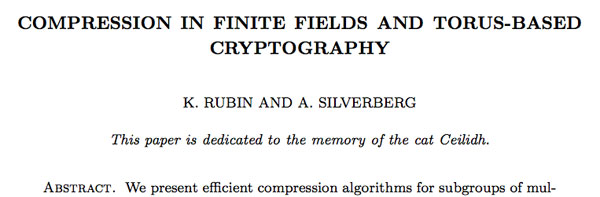Yesterday, Jan Stienstra gave a talk at theARTS entitled “Quivers, superpotentials and Dimer Models”. He started off by telling that the talk was based on a paper he put on the arXiv Hypergeometric Systems in two Variables, Quivers, Dimers and Dessins d’Enfants but that he was not going to say a thing about dessins but would rather focuss on the connection with superpotentials instead…pleasing some members of the public, while driving others to utter despair.

Anyway, it gave me the opportunity to figure out for myself what dessins might have to do with dimers, whathever these beasts are. Soon enough he put on a slide containing the definition of a dimer and from that moment on I was lost in my own thoughts… realizing that a dessin d’enfant had to be a dimer for the Dedekind tessellation of its associated Riemann surface!
and a few minutes later I could slap myself on the head for not having thought of this before :

There is a natural way to associate to a Farey symbol (aka a permutation representation of the modular group) a quiver and a superpotential (aka a necklace) defining (conjecturally) a Calabi-Yau algebra! Moreover, different embeddings of the cuboid tree diagrams in the hyperbolic plane may (again conjecturally) give rise to all sorts of arty-farty fanshi-wanshi dualities…

I’ll give here the details of the simplest example I worked out during the talk and will come back to general procedure later, when I’ve done a reference check. I don’t claim any originality here and probably all of this is contained in Stienstra’s paper or in some physics-paper, so if you know of a reference, please leave a comment. Okay, remember the Dedekind tessellation ?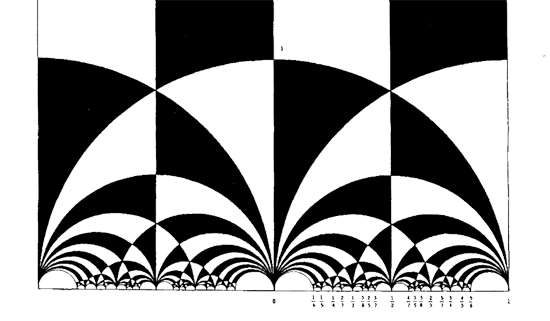So, all hyperbolic triangles we will encounter below are colored black or white. Now, take a Farey symbol and consider its associated special polygon in the hyperbolic plane. If we start with the Farey symbol

$$\xymatrix{\infty \ar@{-}_{(1)}[r] & 0 \ar@{-}_{\bullet}[r] & 1 \ar@{-}_{(1)}[r] & \infty}$$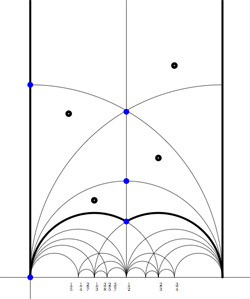we get the special polygonal region bounded by the thick edges, the vertical edges are identified as are the two bottom edges. Hence, this fundamental domain has 6 vertices (the 5 blue dots and the point at $i \infty$) and 8 hyperbolic triangles (4 colored black, indicated by a black dot, and 4 white ones).

Right, now let us associate a quiver to this triangulation (which embeds the quiver in the corresponding Riemann surface). The vertices of the triangulation are also the vertices of the quiver (so in our case we are going for a quiver with 6 vertices). Every hyperbolic edge in the triangulation gives one arrow in the quiver between the corresponding vertices. The orientation of the arrow is determined by the color of a triangle of which it is an edge : if the triangle is black, we run around its edges counter-clockwise and if the triangle is white we run over its edges clockwise (that is, the orientation of the arrow is independent of the choice of triangles to determine it). In our example, there is one arrows directed from the vertex at $i$ to the vertex at $0$, whether you use the black triangle on the left to determine the orientation or the white triangle on the right. If we do this for all edges in the triangulation we arrive at the quiver below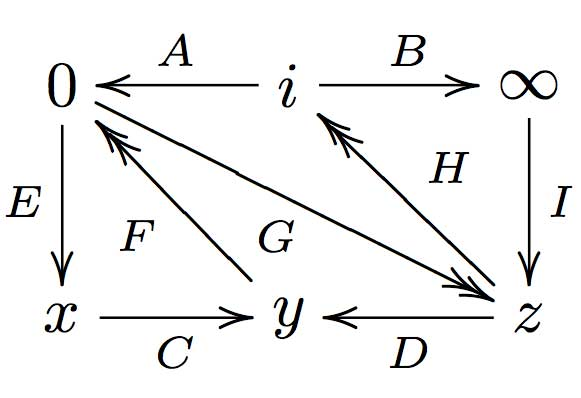where x,y and z are the three finite vertices on the $\frac{1}{2}$-axis from bottom to top and where I’ve used the physics-convention for double arrows, that is there are two F-arrows, two G-arrows and two H-arrows. Observe that the quiver is of Calabi-Yau type meaning that there are as much arrows coming into a vertex as there are arrows leaving the vertex.

Now that we have our quiver we determine the superpotential as follows. Fix an orientation on the Riemann surface (for example counter-clockwise) and sum over all black triangles the product of the edge-arrows counterclockwise MINUS sum over all white triangles
the product of the edge arrows counterclockwise. So, in our example we have the cubic superpotential

$IH’B+HAG+G’DF+FEC-BHI-H’G’A-GFD-CEF’$

From this we get the associated noncommutative algebra, which is the quotient of the path algebra of the above quiver modulo the following ‘commutativity relations’

$\begin{cases} GH &=G’H’ \\ IH’ &= IH \\ FE &= F’E \\ F’G’ &= FG \\ CF &= CF’ \\ EC &= GD \\ G’D &= EC \\ HA &= DF \\ DF’ &= H’A \\ AG &= BI \\ BI &= AG’ \end{cases}$

and morally this should be a Calabi-Yau algebra (( can someone who knows more about CYs verify this? )). This concludes the walk through of the procedure. Summarizing : to every Farey-symbol one associates a Calabi-Yau quiver and superpotential, possibly giving a Calabi-Yau algebra!